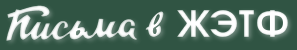HomeFor authorsSubmission statusArchiveArchive (English)Current Volume 114 Volume 113 Volume 112 Volume 111 Volume 110 Volume 109 Volume 108 Volume 107 Volume 106 Volume 105 Volume 104 Volume 103 Volume 102 Volume 101 Volume 100 Volume 99 Volume 98 Volume 97 Volume 96 Volume 95 Volume 94 Volume 93SearchVOLUME 100 | ISSUE 5 | PAGE 323
Final state interaction effects in B+→ D+K0 decay
Abstract
In this research the exclusive decay of B+→ D+K0 is calculated by QCD factorization method (QCDF) and final state interaction (FSI). First, the B+→ D+K0 decay is calculated via QCDF method. The result that is found by using the QCDF method is less than the experimental result. So FSI is considered to solve the B+→ D+K0 decay. For this decay, the Ds+π0, Ds+*ρ0, Ds+*φ via the exchange of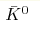,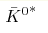, D-, and D-* mesons are chosen for the intermediate states. The above intermediate states are calculated by using the QCDF method. In the FSI effects, the results of our calculations depend on η as the phenomenological parameter. The range of this parameter is selected from 2 to 2.4. It is found that if η=2.4 is selected, the numbers of the branching ratio are placed in the experimental range. The experimental branching ratio of this decay is less than 2.9•10-6 and our results calculated by QCDF and FSI are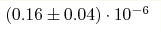and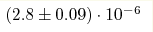respectively.